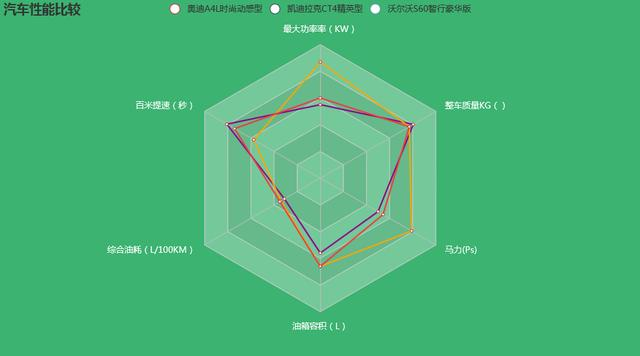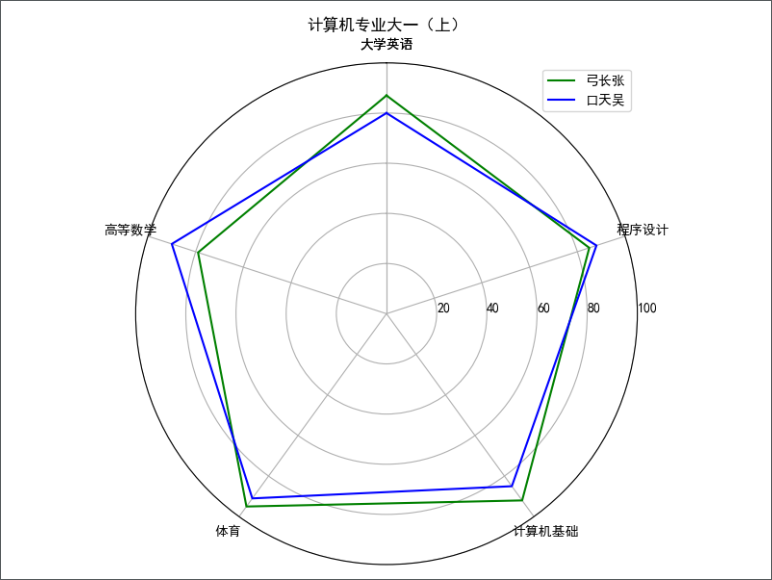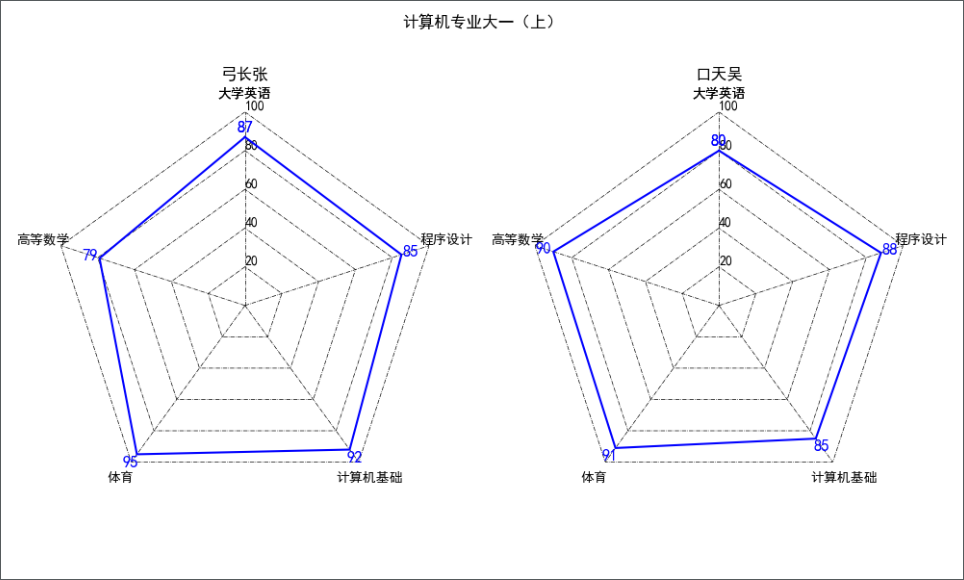•shell
• 雷达图组件雷达图组件雷达图组件雷达图组件雷达图组件雷达图组件
• 这是一款整理发布的雷达图分析法，适用于公司企业营销人员学习参考雷达图分析法，进而更好提升...该文档为雷达图分析法，是一份很不错的参考资料，具有较高参考价值，感兴趣的可以下载看看
• 雷达图是以从同一点开始的轴上表示的三个或更多个定量变量的二维图表的形式显示多变量数据的图形方法,雷达图通常用于综合分析多个指标，具有完整，清晰和直观的优点。下面以实际例子给大家讲解一下雷达图的应用场景...
雷达图是以从同一点开始的轴上表示的三个或更多个定量变量的二维图表的形式显示多变量数据的图形方法,雷达图通常用于综合分析多个指标，具有完整，清晰和直观的优点。下面以实际例子给大家讲解一下雷达图的应用场景和绘制方法：一、比较汽车性能这类雷达图一般用于比较同类事物不同纬度性能的优劣，以奥迪A4L时尚动感型和凯迪拉克CT4精英型为例，我们来画一下这两种汽车的雷达图，代码如下：import pyecharts.options as optsfrom pyecharts.charts import Radarv1 = [[110, 9.7, 6.2, 56, 150, 1610]]v2 = [[174, 6.9, 6.8, 66, 237, 1540]]c=(    Radar(init_opts=opts.InitOpts(bg_color="#3CB371"))   #设置背景颜色    .add_schema(        schema=[            opts.RadarIndicatorItem(name="最大功率率(KW)", max_=200),            opts.RadarIndicatorItem(name="百米提速(秒)", max_=12),            opts.RadarIndicatorItem(name="综合油耗(L/100KM)", max_=20),            opts.RadarIndicatorItem(name="油箱容积(L)", max_=100),            opts.RadarIndicatorItem(name="马力(Ps)", max_=300),            opts.RadarIndicatorItem(name="整车质量KG()", max_=2000),        ],        splitarea_opt=opts.SplitAreaOpts(            is_show=True, areastyle_opts=opts.AreaStyleOpts(opacity=1)  #是否显示分隔区域，透明度设置为1        ),        textstyle_opts=opts.TextStyleOpts(color="#fff"),    )    .add(        series_name="奥迪A4L时尚动感型",        data=v1,        linestyle_opts=opts.LineStyleOpts(color="#8B008B",width=2),   #线的颜色、宽度    )    .add(        series_name="凯迪拉克CT4精英型",        data=v2,        linestyle_opts=opts.LineStyleOpts(color="#FFA500",width=2),   #线的颜色、宽度    )    .set_series_opts(label_opts=opts.LabelOpts(is_show=False))  #不显示数字    .set_global_opts(        title_opts=opts.TitleOpts(), legend_opts=opts.LegendOpts()    ))c.render_notebook()参数介绍：1.通过设置InitOpts的bg_color参数，可以改变背景颜色2.通过设置add_schema的schema参数，可以添加更多纬度变量3.通过设置LineStyleOpts的color参数，可以设置线的颜色和宽度通过雷达图，可以清晰的比较两种汽车性能指标的好坏，非常直观如果感觉两台车不过瘾，我们可以再加1台：二、比较不同城市近期天气状况from pyecharts import options as optsfrom pyecharts.charts import Radarvalue_bj = [    [55, 9, 56, 0.46, 18, 6, 1],    [25, 11, 21, 0.65, 34, 9, 2],    [56, 7, 63, 0.3, 14, 5, 3],    [33, 7, 29, 0.33, 16, 6, 4],    [42, 24, 44, 0.76, 40, 16, 5],    [82, 58, 90, 1.77, 68, 33, 6],    [74, 49, 77, 1.46, 48, 27, 7],    [78, 55, 80, 1.29, 59, 29, 8],    [267, 216, 280, 4.8, 108, 64, 9],    [185, 127, 216, 2.52, 61, 27, 10],    [39, 19, 38, 0.57, 31, 15, 11],    [41, 11, 40, 0.43, 21, 7, 12],]value_sh = [    [91, 45, 125, 0.82, 34, 23, 1],    [65, 27, 78, 0.86, 45, 29, 2],    [83, 60, 84, 1.09, 73, 27, 3],    [109, 81, 121, 1.28, 68, 51, 4],    [106, 77, 114, 1.07, 55, 51, 5],    [109, 81, 121, 1.28, 68, 51, 6],    [106, 77, 114, 1.07, 55, 51, 7],    [89, 65, 78, 0.86, 51, 26, 8],    [53, 33, 47, 0.64, 50, 17, 9],    [80, 55, 80, 1.01, 75, 24, 10],    [117, 81, 124, 1.03, 45, 24, 11],    [99, 71, 142, 1.1, 62, 42, 12],]c_schema = [    {"name": "AQI", "max": 300, "min": 5},    {"name": "PM2.5", "max": 250, "min": 20},    {"name": "PM10", "max": 300, "min": 5},    {"name": "CO", "max": 5},    {"name": "NO2", "max": 200},    {"name": "SO2", "max": 100},]c = (    Radar(init_opts=opts.InitOpts(bg_color="#8B658B"))    .add_schema(schema=c_schema, shape="polygon")    .add("北京", value_bj,color="#8B008B",linestyle_opts=opts.LineStyleOpts(width=2))    .add("上海", value_sh,color="#FF4500",linestyle_opts=opts.LineStyleOpts(width=2))    .set_series_opts(label_opts=opts.LabelOpts(is_show=False))    .set_global_opts(title_opts=opts.TitleOpts()))c.render_notebook()通过增加数据种类，可以比较同一纬度、不同时间下的差距，如上图，通过展示北京、上海两座城市12天的天气情况，可以清晰的看出上海的天气要比北京好。-完-完整代码请关注公众号【python数据分析之禅】并回复雷达图获取
展开全文• 将鸢尾花数据python绘制散点图，雷达图，轮廓图，调和曲线图等
• 本篇文章介绍使用matplotlib绘制雷达图雷达图也被称为网络图，蜘蛛图，星图，蜘蛛网图，是一个不规则的多边形。雷达图可以形象地展示相同事物的多维指标，应用场景非常多。一、matplotlib绘制圆形雷达图# coding=...本篇文章介绍使用matplotlib绘制雷达图。雷达图也被称为网络图，蜘蛛图，星图，蜘蛛网图，是一个不规则的多边形。雷达图可以形象地展示相同事物的多维指标，应用场景非常多。一、matplotlib绘制圆形雷达图# coding=utf-8import numpy as npimport matplotlib.pyplot as pltresults = [{"大学英语": 87, "高等数学": 79, "体育": 95, "计算机基础": 92, "程序设计": 85},{"大学英语": 80, "高等数学": 90, "体育": 91, "计算机基础": 85, "程序设计": 88}]data_length = len(results)# 将极坐标根据数据长度进行等分angles = np.linspace(0, 2*np.pi, data_length, endpoint=False)labels = [key for key in results.keys()]score = [[v for v in result.values()] for result in results]# 使雷达图数据封闭score_a = np.concatenate((score, [score]))score_b = np.concatenate((score, [score]))angles = np.concatenate((angles, [angles]))labels = np.concatenate((labels, [labels]))# 设置图形的大小fig = plt.figure(figsize=(8, 6), dpi=100)# 新建一个子图ax = plt.subplot(111, polar=True)# 绘制雷达图ax.plot(angles, score_a, color='g')ax.plot(angles, score_b, color='b')# 设置雷达图中每一项的标签显示ax.set_thetagrids(angles*180/np.pi, labels)# 设置雷达图的0度起始位置ax.set_theta_zero_location('N')# 设置雷达图的坐标刻度范围ax.set_rlim(0, 100)# 设置雷达图的坐标值显示角度，相对于起始角度的偏移量ax.set_rlabel_position(270)ax.set_title("计算机专业大一(上)")plt.legend(["弓长张", "口天吴"], loc='best')plt.show()运行结果：绘制雷达图需要先建立极坐标系，关于极坐标系可以自己了解一下。建立好极坐标系后可以在极坐标系中绘制折线图、柱状图等，大部分情况，都是用折线图，形成一个不规则的闭合多边形。本文中用某高校大一的期末考试成绩作为例子来演示雷达图的效果。linspace(): 用于将极坐标系根据数据的维度进行等分，linspace()函数的第一个参数传入起始角度，第二参数传入结束角度，第三个参数传入分成多少等份。其他参数根据需要传入，如endpoint默认为True，最后一个数据处于结束的角度，根据本例中前面的参数0~2π，应该设置为False，否则最后一个数据与第一个数据角度重叠了。concatenate(): 使雷达图的数据是环形封闭的，concatenate()函数的第一个参数是一个元组，元组中的每个元素是一个数组，concatenate()函数将这些数组连接到一起，组成一个新的数组。要让绘制的雷达图封闭，将数据的第一个值连接到数组的结尾即可。本文用折线图plot()来绘制雷达图，使用figure()函数设置好图形的大小和清晰度，然后使用subplot()函数来创建一张子图。subplot()函数的第一个参数传入长度为3的数字，第一个数字表示将画布分成几行，第二个数字表示将画布分成几列，第三个数字表示当前的子图处于哪个位置(按从左至右、从上到下的顺序排序)，第三个数字不能超出前两个数字切分的子图数范围。如111表示将画布分成一行一列(只有一张子图)，当前的子图处于第一张子图中。在subplot()函数中，将polar参数设置成True，得到的图形才是极坐标。极坐标系设置完成后，使用子图对象ax调用折线图函数plot()，即可绘出雷达图。如果有多组数据，多次调用plot()函数即可。使用set_thetagrids()方法设置雷达图中每个维度的标签和显示位置。使用set_theta_zero_location()方法设置雷达图的0度位置，可以传入"N"、"NW"、"W"、"SW"、"S"、"SE"、"E"、"NE"八个方位缩写。使用set_rlim()方法设置极坐标上的刻度范围。使用set_rlabel_position()方法设置极坐标上的刻度标签显示位置，传入一个相对于雷达图0度的角度值。当然还可以根据需要设置其他属性，如标题、图例等。在上面的例子中，将两位同学的考试成绩绘制成了雷达图，通过雷达图，可以看出两个人的单科成绩互有高低，而整体来看，两位同学的成绩都很优秀。上面的雷达图中，网格线都是圆形的，而用折线图连接的雷达图两个维度之间是直线连接的，所以将网格线换成多边形会更合理一点。二、matplotlib绘制多边形雷达图import numpy as npimport matplotlib.pyplot as pltresults = [{"大学英语": 87, "高等数学": 79, "体育": 95, "计算机基础": 92, "程序设计": 85},{"大学英语": 80, "高等数学": 90, "体育": 91, "计算机基础": 85, "程序设计": 88}]data_length = len(results)angles = np.linspace(0, 2*np.pi, data_length, endpoint=False)labels = [key for key in results.keys()]score = [[v for v in result.values()] for result in results]score_a = np.concatenate((score, [score]))score_b = np.concatenate((score, [score]))angles = np.concatenate((angles, [angles]))labels = np.concatenate((labels, [labels]))fig = plt.figure(figsize=(10, 6), dpi=100)fig.suptitle("计算机专业大一(上)")ax1 = plt.subplot(121, polar=True)ax2 = plt.subplot(122, polar=True)ax, data, name = [ax1, ax2], [score_a, score_b], ["弓长张", "口天吴"]for i in range(2):for j in np.arange(0, 100+20, 20):ax[i].plot(angles, 6*[j], '-.', lw=0.5, color='black')for j in range(5):ax[i].plot([angles[j], angles[j]], [0, 100], '-.', lw=0.5, color='black')ax[i].plot(angles, data[i], color='b')# 隐藏最外圈的圆ax[i].spines['polar'].set_visible(False)# 隐藏圆形网格线ax[i].grid(False)for a, b in zip(angles, data[i]):ax[i].text(a, b+5, '%.00f' % b, ha='center', va='center', fontsize=12, color='b')ax[i].set_thetagrids(angles*180/np.pi, labels)ax[i].set_theta_zero_location('N')ax[i].set_rlim(0, 100)ax[i].set_rlabel_position(0)ax[i].set_title(name[i])plt.show()运行结果：在极坐标系中，极径值相等的点在一个圆上，所以绘制的雷达图中，网格线默认都是圆形的。如果要绘制多边形的雷达图，则需要将圆形的网格线隐藏，然后根据刻度范围绘制出多边形的网格线。首先使用plot()函数，根据刻度范围，绘制出同心的多个多边形和多个维度方向的极轴，作为雷达图的网格线，形成一张“网”。链式调用极坐标的spines['polar'].set_visible()方法，传入参数False，将极坐标系最外圈的圆形隐藏。调用grid()方法，传入参数False，将极坐标系中的的圆形网格线隐藏。修改完网格线后，即可达到多边形的效果。在第二次绘制雷达图时，将两位同学的成绩分到两张不同的雷达图中，并用text()方法设置了每个维度的数据标注，使用fig对象的suptitle()方法来设置整张图形的标题。上面的两次绘图，将两位同学的成绩绘制在同一张雷达图时，更方便对比两位同学的成绩，如比较谁更全面、更优秀。分开绘制时，更方便分析个人的成绩情况，如是否偏科。而相对于圆形的雷达图，在多边形的雷达图中，不会出现雷达图与网格线的不合理交叉(雷达图与网格线交叉两次)，使用多边形网格线更合理。
展开全文• 雷达图-pyecharts蛛网图，最早知道是在玩FIFA游戏的时候，球员的能力用蛛网图来表示与比较，那时觉得非常新鲜。后来，在实际的工作中，其实很少用到：一方面，直接提供蛛网图的工具少；另一方面，过往的经历中多维度...雷达图-pyecharts蛛网图，最早知道是在玩FIFA游戏的时候，球员的能力用蛛网图来表示与比较，那时觉得非常新鲜。后来，在实际的工作中，其实很少用到：一方面，直接提供蛛网图的工具少；另一方面，过往的经历中多维度比较用到的地方也比较少。在学习Python的过程中，重新燃起对雷达图的兴趣，但在python重要的图库Matplotlib与Seaborn里都没有直接实现雷达图的函数。因此，虽然感兴趣，也没有去触及，直到一天在Udacity数据分析进阶VIP班的一个学生的Tableau作业里看到他使用了雷达图（Tableau图库里也没有直接提供雷达图），使我饶有兴趣地去思考雷达图到底是什么？最初的想法最初的想法，在直角坐标系通过画圆的方式寻找多边形在圆轴上点的坐标，只要知道半径长度，以及原点与圆周上的点的连线与X轴的夹角便能通过三角函数求出x与y 的坐标。向量.png半径可看作是一个向量。向量是一个方向，向量中的每一个元素代表着维度，维度的值是维度方向的距离。直角坐标系由x轴，y轴及原点组成的二维空间，x是一个维度，x的值在x轴方向上与原点之间的距离，y是另一个维度，同样的，y的值则是在y轴方向上与原点的距离，x与y平行移动的交汇点为坐标，坐标点与原点的连线是x轴与y轴上的两个两个向量的相加所形成的新向量，暂命名为A向量，A向量的长度为r，围绕着原点旋转便能形成一个以原点为圆心，r为半径的圆，根据A向量与x轴行程的角度总能得出A向量在x轴上的长度为，在y轴上的长度为，由此，可以得出该在圆上的任意一个点的坐标(x,y)。假设雷达图由6个维度构成，那么最初躺在x轴上的向量相当于旋转了6次，每次旋转60º在圆周上留下一个点，点与点之间的连线构成了雷达图。那么，使用折线图便能实现雷达图。突然醒悟仔细翻读Matplotlib的范例，看到了polar坐标系，突然想明白：为什么不直接旋转X坐标轴呢？将直角坐标系中的X轴首尾相连绕成了一个圆，将x轴的线性的长度转换成了弧长，Y轴不变，在此之下的折线图就是雷达图，仅此而已。想明白了，生成雷达图便成了轻而易举之事。接下来，将详细解释雷达图编写的过程。用Python绘制雷达图pyechart用python语言制作了一系列流行的图，其中包括雷达图。我将其雷达图的截图放在了本文的最顶端。然而pyechart只是用Python语言生成的Java，应用在html里。当然，在实际应用中，用网页显示与观众的互动性更强，如果用网页作为仪表盘，显然使用pyechart就好了。但在这里，我期望去了解雷达图的原理，因此借用pyechart-雷达图示例中的数据，用Matplotlib  来重现。用Matplotlib 绘制的雷达图这是使用Matplotlib画出的结果，除了不会动态显示数据以外，长相与pyechart的雷达图基本保持了一致，过程并不复杂。接下来，将分步骤展现实现的过程与代码。Python分步骤实现雷达图1. 范例数据schema = [("销售", 6000),("管理", 16000),("信息技术", 30000),("客户服务", 38000),("研发", 52000),("市场", 25000)]v1 = [[4300, 10000, 28000, 35000, 50000, 19000]]v2 = [[5000, 14000, 28000, 31000, 42000, 21000]]schema 中包含标签与6个维度分别的总分，v1、v2可能是2个部门分别按6个维度打分的结果。整理数据，首先抽出labels， 然后对v1、v2 进行归一(标准化，normalize)，将分值转化为比率，或百分制。# 拆分schema到标签labs与总分full_markslabs = []full_marks = []for value in schema:labs.append(value)full_marks.append(value)# v1,v2进行归一处里Y = np.vstack((v1,v2))Y = Y/np.array(full_marks)print('labs = {}'.format(labs))print('Y = {}'.format(Y))--------------------------------------------------------------------------Output:labs = ['销售', '管理', '信息技术', '客户服务', '研发', '市场']Y = [[ 0.71666667  0.625       0.93333333  0.92105263  0.96153846  0.76      ][ 0.83333333  0.875       0.93333333  0.81578947  0.80769231  0.84      ]]2. X轴变形x轴上所呈现的应该是labs，用labs的index来作为x轴上的坐标，由于x轴将由一根直线首尾相接成圆，原先x轴上的点离原点的距离将相应地换算为弧长，每一维度间所间隔的角度由维度决定，将圆周长切分成个等份的弧度。弧长的计算公式为:# 获取 r 与 thetaN = len(labs)r = np.arange(N)theta = np.linspace(0, 360, N, endpoint=False)# 将角度转化为单位弧度X_ticks = np.radians(theta) # x轴标签所在的位置print(' N = {:d},
r = {},
theta = {},
X_ticks = {}'.format(N, r, theta, X_ticks))-------------------------------------------------------------------Output:N = 6,r = [0 1 2 3 4 5],theta = [   0.   60.  120.  180.  240.  300.],X_ticks = [ 0.      1.0472  2.0944  3.1416  4.1888  5.236 ]3. 制图1简单地转换一下X轴的数值，然后设置坐标系为"polar”，雷达图应该就能被制作出来了。fig, ax = plt.subplots(subplot_kw=dict(projection='polar'))ax.plot(X_ticks, Y, marker='o')ax.plot(X_ticks, Y, marker='o')ax.set_xticks(X_ticks)plt.show()首次尝试首次尝试的雷达图有一些问题：联线缺了一个口，需要将数列的第一个数字增补到最后一个，使得首位相连；图像看起来有些歪，正中点在水平线上，尤其是单数维度时。因此需要旋转图像，使得正中点在垂直线上。4. 调整# 获取 r 与 thetaN = len(labs)r = np.arange(N)theta = np.linspace(0, 360, N, endpoint=False)# 调整角度使得正中在垂直线上adj_angle = theta[-1] + 90 - 360theta += adj_angle# 将角度转化为单位弧度X_ticks = np.radians(theta) # x轴标签所在的位置# 首尾相连X = np.append(X_ticks,X_ticks)Y = np.hstack((Y, Y[:,0].reshape(2,1)))print('theta = {},
X = {},
Y={}'.format(theta, X.round(4), Y.round(2)))-------------------------------------------------------------------------Output:theta = [  30.   90.  150.  210.  270.  330.],X = [ 0.5236  1.5708  2.618   3.6652  4.7124  5.7596  0.5236],Y=[[ 0.72  0.62  0.93  0.92  0.96  0.76  0.72][ 0.83  0.88  0.93  0.82  0.81  0.84  0.83]]所有的角度增加了30º，X与Y轴的首尾的数字都已相同，雷达图应该就绪。二次尝试执行与首次制图时相同的代码，只是把X_ticks与Y替换成调整后的X与Y，雷达图的内里已经全部出来了，与pyechart雷达图的图形一致，现在还差一些形状与数据标签。5. 背景制作fig, ax = plt.subplots(figsize=(5, 5),subplot_kw=dict(projection='polar'))# 画图ax.plot(X, Y, marker='o')ax.plot(X, Y, marker='o')ax.set_xticks(X)# 设置背景坐标系ax.set_xticklabels(labs, fontproperties = labFont, fontsize = 'large') # 设置标签ax.set_yticklabels([])ax.spines['polar'].set_visible(False) # 将轴隐藏ax.grid(axis='y') # 只有y轴设置grid# 设置X轴的gridn_grids = np.linspace(0,1, 6, endpoint=True) # grid的网格数grids = [[i] * (len(X)) for i in n_grids] #grids的半径for i, grid in enumerate(grids[:-1]): # 给grid 填充间隔色ax.plot(X, grid, color='grey', linewidth=0.5)if (i>0) & (i % 2 == 0):ax.fill_between(X, grids[i], grids[i-1], color='grey', alpha=0.1)plt.show()接近成功基本上成功了，就差一个轴 (sprine) 的设置，非常抱歉没有弄懂Matplotlib如何去设置轴的形状，不过好在他的范例中给出了雷达图的函数代码，运行这段函数，向figure中的projection注册了这段代码后，将上文的代码中的'polar' 改为'radar'便大功告成了。重写的完整代码如下：# 调用Radar图函数N = len(labs)theta = radar_factory(N, frame='polygon')fig, ax = plt.subplots(figsize=(5, 5),subplot_kw=dict(projection='radar'))# 画图ax.plot(X, Y, marker='o')ax.plot(X, Y, marker='o')ax.set_xticks(X)# 设置背景坐标系ax.set_xticklabels(labs, fontproperties = labFont, fontsize = 'large') # 设置标签ax.set_yticklabels([])ax.spines['polar'].set_visible(False) # 将轴隐藏ax.grid(axis='y') # 只有y轴设置grid# 设置X轴的gridn_grids = np.linspace(0,1, 6, endpoint=True) # grid的网格数grids = [[i] * (len(X)) for i in n_grids] #grids的半径for i, grid in enumerate(grids[:-1]): # 给grid 填充间隔色ax.plot(X, grid, color='grey', linewidth=0.5)if (i>0) & (i % 2 == 0):ax.fill_between(X, grids[i], grids[i-1], color='grey', alpha=0.1)plt.show()结果已在前述展示过了，不再重复。
展开全文• 雷达图-pyecharts蛛网图，最早知道是在玩FIFA游戏的时候，球员的能力用蛛网图来表示与比较，那时觉得非常新鲜。后来，在实际的工作中，其实很少用到：一方面，直接提供蛛网图的工具少；另一方面，过往的经历中多维度...雷达图-pyecharts蛛网图，最早知道是在玩FIFA游戏的时候，球员的能力用蛛网图来表示与比较，那时觉得非常新鲜。后来，在实际的工作中，其实很少用到：一方面，直接提供蛛网图的工具少；另一方面，过往的经历中多维度比较用到的地方也比较少。在学习Python的过程中，重新燃起对雷达图的兴趣，但在python重要的图库Matplotlib与Seaborn里都没有直接实现雷达图的函数。因此，虽然感兴趣，也没有去触及，直到一天在Udacity数据分析进阶VIP班的一个学生的Tableau作业里看到他使用了雷达图（Tableau图库里也没有直接提供雷达图），使我饶有兴趣地去思考雷达图到底是什么？最初的想法最初的想法，在直角坐标系通过画圆的方式寻找多边形在圆轴上点的坐标，只要知道半径长度，以及原点与圆周上的点的连线与X轴的夹角便能通过三角函数求出x与y 的坐标。向量.png半径可看作是一个向量。向量是一个方向，向量中的每一个元素代表着维度，维度的值是维度方向的距离。直角坐标系由x轴，y轴及原点组成的二维空间，x是一个维度，x的值在x轴方向上与原点之间的距离，y是另一个维度，同样的，y的值则是在y轴方向上与原点的距离，x与y平行移动的交汇点为坐标，坐标点与原点的连线是x轴与y轴上的两个两个向量的相加所形成的新向量，暂命名为A向量，A向量的长度为r，围绕着原点旋转便能形成一个以原点为圆心，r为半径的圆，根据A向量与x轴行程的角度总能得出A向量在x轴上的长度为，在y轴上的长度为，由此，可以得出该在圆上的任意一个点的坐标(x,y)。假设雷达图由6个维度构成，那么最初躺在x轴上的向量相当于旋转了6次，每次旋转60º在圆周上留下一个点，点与点之间的连线构成了雷达图。那么，使用折线图便能实现雷达图。突然醒悟仔细翻读Matplotlib的范例，看到了polar坐标系，突然想明白：为什么不直接旋转X坐标轴呢？将直角坐标系中的X轴首尾相连绕成了一个圆，将x轴的线性的长度转换成了弧长，Y轴不变，在此之下的折线图就是雷达图，仅此而已。想明白了，生成雷达图便成了轻而易举之事。接下来，将详细解释雷达图编写的过程。用Python绘制雷达图pyechart用python语言制作了一系列流行的图，其中包括雷达图。我将其雷达图的截图放在了本文的最顶端。然而pyechart只是用Python语言生成的Java，应用在html里。当然，在实际应用中，用网页显示与观众的互动性更强，如果用网页作为仪表盘，显然使用pyechart就好了。但在这里，我期望去了解雷达图的原理，因此借用pyechart-雷达图示例中的数据，用Matplotlib  来重现。用Matplotlib 绘制的雷达图这是使用Matplotlib画出的结果，除了不会动态显示数据以外，长相与pyechart的雷达图基本保持了一致，过程并不复杂。接下来，将分步骤展现实现的过程与代码。Python分步骤实现雷达图1. 范例数据schema = [("销售", 6000),("管理", 16000),("信息技术", 30000),("客户服务", 38000),("研发", 52000),("市场", 25000)]v1 = [[4300, 10000, 28000, 35000, 50000, 19000]]v2 = [[5000, 14000, 28000, 31000, 42000, 21000]]schema 中包含标签与6个维度分别的总分，v1、v2可能是2个部门分别按6个维度打分的结果。整理数据，首先抽出labels， 然后对v1、v2 进行归一(标准化，normalize)，将分值转化为比率，或百分制。# 拆分schema到标签labs与总分full_markslabs = []full_marks = []for value in schema:labs.append(value)full_marks.append(value)# v1,v2进行归一处里Y = np.vstack((v1,v2))Y = Y/np.array(full_marks)print('labs = {}'.format(labs))print('Y = {}'.format(Y))--------------------------------------------------------------------------Output:labs = ['销售', '管理', '信息技术', '客户服务', '研发', '市场']Y = [[ 0.71666667  0.625       0.93333333  0.92105263  0.96153846  0.76      ][ 0.83333333  0.875       0.93333333  0.81578947  0.80769231  0.84      ]]2. X轴变形x轴上所呈现的应该是labs，用labs的index来作为x轴上的坐标，由于x轴将由一根直线首尾相接成圆，原先x轴上的点离原点的距离将相应地换算为弧长，每一维度间所间隔的角度由维度决定，将圆周长切分成个等份的弧度。弧长的计算公式为:# 获取 r 与 thetaN = len(labs)r = np.arange(N)theta = np.linspace(0, 360, N, endpoint=False)# 将角度转化为单位弧度X_ticks = np.radians(theta) # x轴标签所在的位置print(' N = {:d},
r = {},
theta = {},
X_ticks = {}'.format(N, r, theta, X_ticks))-------------------------------------------------------------------Output:N = 6,r = [0 1 2 3 4 5],theta = [   0.   60.  120.  180.  240.  300.],X_ticks = [ 0.      1.0472  2.0944  3.1416  4.1888  5.236 ]3. 制图1简单地转换一下X轴的数值，然后设置坐标系为“polar”，雷达图应该就能被制作出来了。fig, ax = plt.subplots(subplot_kw=dict(projection='polar'))ax.plot(X_ticks, Y, marker='o')ax.plot(X_ticks, Y, marker='o')ax.set_xticks(X_ticks)plt.show()首次尝试首次尝试的雷达图有一些问题：联线缺了一个口，需要将数列的第一个数字增补到最后一个，使得首位相连；图像看起来有些歪，正中点在水平线上，尤其是单数维度时。因此需要旋转图像，使得正中点在垂直线上。4. 调整# 获取 r 与 thetaN = len(labs)r = np.arange(N)theta = np.linspace(0, 360, N, endpoint=False)# 调整角度使得正中在垂直线上adj_angle = theta[-1] + 90 - 360theta += adj_angle# 将角度转化为单位弧度X_ticks = np.radians(theta) # x轴标签所在的位置# 首尾相连X = np.append(X_ticks,X_ticks)Y = np.hstack((Y, Y[:,0].reshape(2,1)))print('theta = {},
X = {},
Y={}'.format(theta, X.round(4), Y.round(2)))-------------------------------------------------------------------------Output:theta = [  30.   90.  150.  210.  270.  330.],X = [ 0.5236  1.5708  2.618   3.6652  4.7124  5.7596  0.5236],Y=[[ 0.72  0.62  0.93  0.92  0.96  0.76  0.72][ 0.83  0.88  0.93  0.82  0.81  0.84  0.83]]所有的角度增加了30º，X与Y轴的首尾的数字都已相同，雷达图应该就绪。二次尝试执行与首次制图时相同的代码，只是把X_ticks与Y替换成调整后的X与Y，雷达图的内里已经全部出来了，与pyechart雷达图的图形一致，现在还差一些形状与数据标签。5. 背景制作fig, ax = plt.subplots(figsize=(5, 5),subplot_kw=dict(projection='polar'))# 画图ax.plot(X, Y, marker='o')ax.plot(X, Y, marker='o')ax.set_xticks(X)# 设置背景坐标系ax.set_xticklabels(labs, fontproperties = labFont, fontsize = 'large') # 设置标签ax.set_yticklabels([])ax.spines['polar'].set_visible(False) # 将轴隐藏ax.grid(axis='y') # 只有y轴设置grid# 设置X轴的gridn_grids = np.linspace(0,1, 6, endpoint=True) # grid的网格数grids = [[i] * (len(X)) for i in n_grids] #grids的半径for i, grid in enumerate(grids[:-1]): # 给grid 填充间隔色ax.plot(X, grid, color='grey', linewidth=0.5)if (i>0) & (i % 2 == 0):ax.fill_between(X, grids[i], grids[i-1], color='grey', alpha=0.1)plt.show()接近成功基本上成功了，就差一个轴 (sprine) 的设置，非常抱歉没有弄懂Matplotlib如何去设置轴的形状，不过好在他的范例中给出了雷达图的函数代码，运行这段函数，向figure中的projection注册了这段代码后，将上文的代码中的'polar' 改为'radar'便大功告成了。重写的完整代码如下：# 调用Radar图函数N = len(labs)theta = radar_factory(N, frame='polygon')fig, ax = plt.subplots(figsize=(5, 5),subplot_kw=dict(projection='radar'))# 画图ax.plot(X, Y, marker='o')ax.plot(X, Y, marker='o')ax.set_xticks(X)# 设置背景坐标系ax.set_xticklabels(labs, fontproperties = labFont, fontsize = 'large') # 设置标签ax.set_yticklabels([])ax.spines['polar'].set_visible(False) # 将轴隐藏ax.grid(axis='y') # 只有y轴设置grid# 设置X轴的gridn_grids = np.linspace(0,1, 6, endpoint=True) # grid的网格数grids = [[i] * (len(X)) for i in n_grids] #grids的半径for i, grid in enumerate(grids[:-1]): # 给grid 填充间隔色ax.plot(X, grid, color='grey', linewidth=0.5)if (i>0) & (i % 2 == 0):ax.fill_between(X, grids[i], grids[i-1], color='grey', alpha=0.1)plt.show()结果已在前述展示过了，不再重复。
展开全文• 自绘雷达图，简单实用，在VC环境绘制雷达图，抛砖引玉。
• 在QT5.9 实现雷达图 和余晖扫描，可以添加，删除卫星，修改位置，颜色，标签，状态，增加卫星间连线，可设置线的颜色，状态；内附说明文档一份
• 安卓雷达图，类似支付宝芝麻分解读，淘宝快递的综合对比图，王者荣耀对战资料图，各种信用评分图示等多边形图表效果。
• 雷达图是什么呢？雷达图又被称为蜘蛛网图，能够显示出多个维度的数据。以中心一点向各个数据方向外发射轴线，且每个数据点的夹角相等、刻度相同，连接每个变量的数据点后就会形成一条多边形。雷达图适用于查看参数...
• Echarts笔记——雷达图（1）radar雷达图坐标系 1.雷达图类型 （ radar ）。 2. 区别以往的直角坐标系，雷达图没有x轴和y轴，使用只适用雷达图雷达图坐标系组件 ：radar 。 3. 需要先配置 radar 雷达坐标系，...数据可视化 echarts js
• ## 雷达图(蜘蛛网图)

千次下载 热门讨论 2015-12-03 17:18:10
最近因为项目需求，要实现一款雷达图来表示用户的各种成就值 雷达图的绘制很简单，只要思路清晰按部就班的绘制就可以了，其中使用得最多，是**路径path类**的使用，使用这个类可以让我们更加方便地绘制出**正多边形*...
• 本篇文章介绍使用matplotlib绘制雷达图雷达图也被称为网络图，蜘蛛图，星图，蜘蛛网图，是一个不规则的多边形。雷达图可以形象地展示相同事物的多维指标，雷达图几乎随处可见，应用场景非常多。一、matplotlib绘制...
• 假设某天某地每三个小时取样的气温为 0时 3时 6时 9时 12时 15时 18时 21时 24时3℃ 5℃ 6℃ 3℃ 1℃ 3℃ 3℃ 2℃ 3℃ 针对温度变化趋势绘制雷达图：代码如下：import numpy as np import matplotlib.pyplot...
• 最近经常用到python做雷达图，现在把相关代码记录下来以备以后查看。（一）在雷达图上表示一个点import matplotlib.pyplot as pltimport numpy as npplt.polar(0.25*np.pi,20,"ro",lw=2)plt.ylim(0,100)plt.show()......